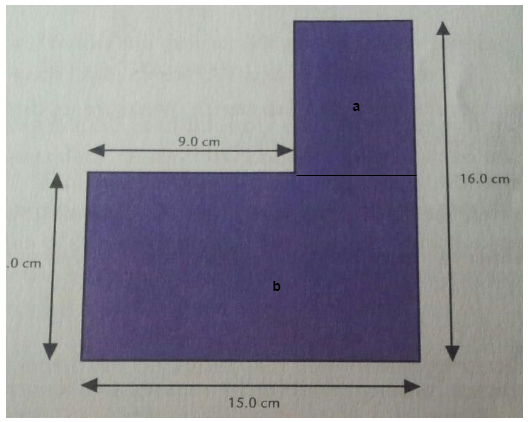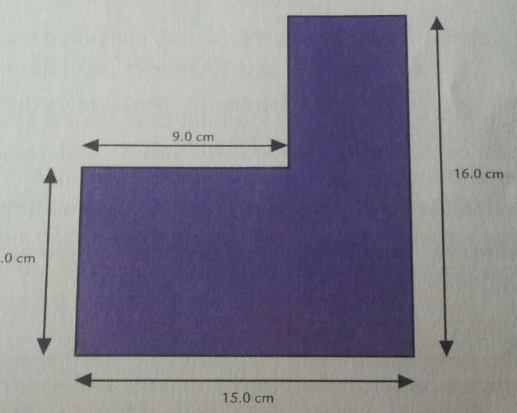# Problem: Calculate the center of mass of the object below. Assume the mass is uniformly distributed.

###### FREE Expert Solution

Consider the figureCenter of mass,

M = Aσ

Ma = (16-8)(6)σ = (48 cm2

Mb = (15)(8.0)σ = (120 cm2

96% (314 ratings)###### Problem Details

Calculate the center of mass of the object below. Assume the mass is uniformly distributed.